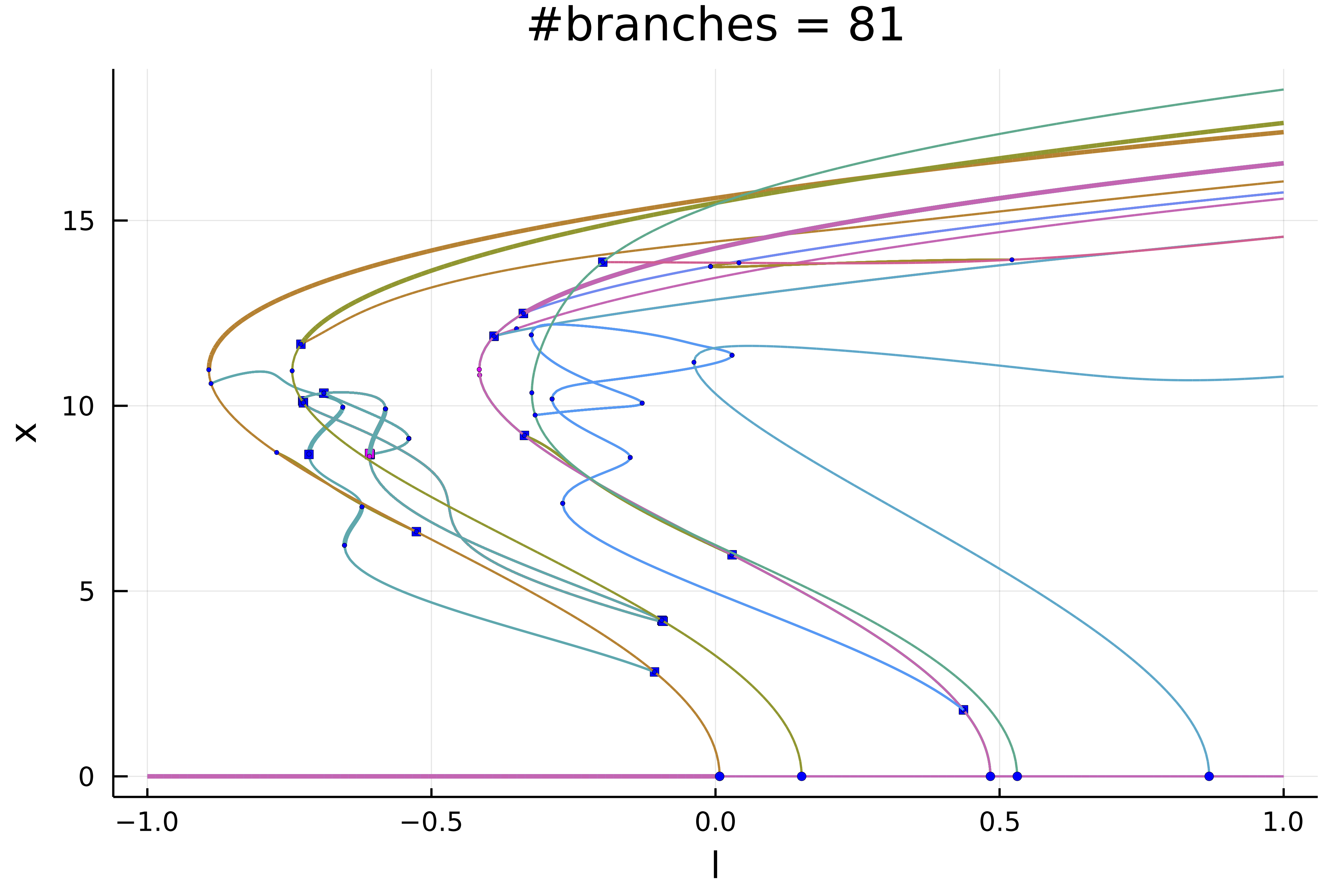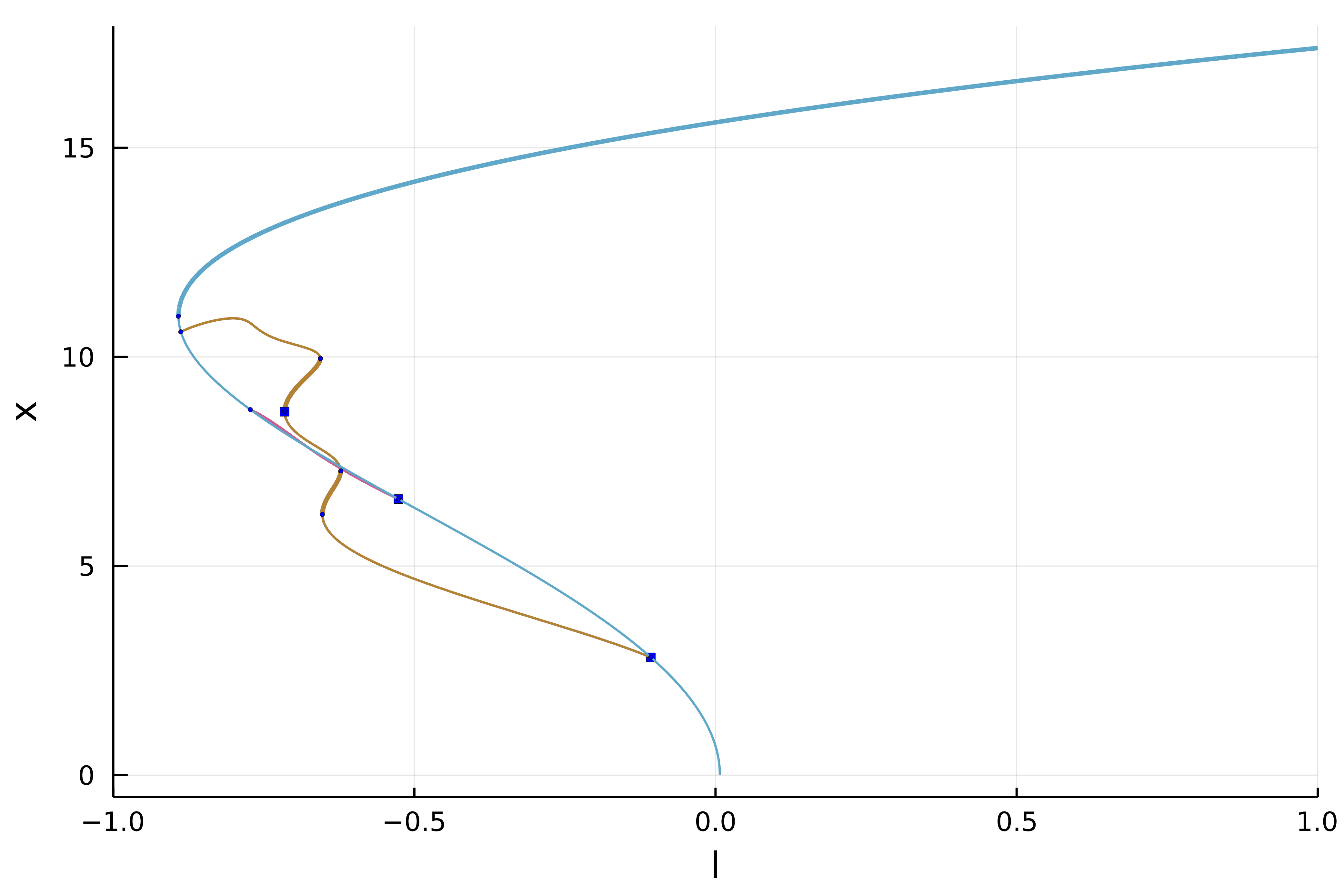# 1d Swift-Hohenberg equation (Automatic)

In this tutorial, we will see how to compute automatically the bifurcation diagram of the 1d Swift-Hohenberg equation

$$$-(I+\Delta)^2 u+l\cdot u +\nu u^3-u^5 = 0\tag{E}$$$

with Dirichlet boundary conditions. We use a Sparse Matrix to express the operator $L_1=(I+\Delta)^2$. We start by loading the packages:

using Revise
using SparseArrays, LinearAlgebra, DiffEqOperators, Setfield, Parameters
using BifurcationKit
using Plots
const BK = BifurcationKit

We then define a discretization of the problem

# define a norm
norminf(x) = norm(x, Inf64)
const _weight = rand(Nx)
normweighted(x) = norm(_weight .* x)

# discretisation
Nx = 200; Lx = 6.;
X = -Lx .+ 2Lx/Nx*(0:Nx-1) |> collect
hx = X-X

# boundary condition
Q = Dirichlet0BC(hx |> typeof)
Dxx = sparse(CenteredDifference(2, 2, hx, Nx) * Q)
Lsh = -(I + Dxx)^2

# functional of the problem
function R_SH(u, par)
@unpack l, ν, L1 = par
out = similar(u)
out .= L1 * u .+ l .* u .+ ν .* u.^3 - u.^5
end

# Jacobian of the function
Jac_sp(u, par) = par.L1 + spdiagm(0 => par.l .+ 3 .* par.ν .* u.^2 .- 5 .* u.^4)

# second derivative
d2R(u,p,dx1,dx2) = @. p.ν * 6u*dx1*dx2 - 5*4u^3*dx1*dx2

# third derivative
d3R(u,p,dx1,dx2,dx3) = @. p.ν * 6dx3*dx1*dx2 - 5*4*3u^2*dx1*dx2*dx3

# parameters associated with the equation
parSH = (l = -0.7, ν = 2., L1 = Lsh)

# initial condition
sol0 = zeros(Nx)

# Bifurcation Problem
prob = BifurcationProblem(R_SH, sol0, parSH, (@lens _.l); J = Jac_sp,
recordFromSolution = (x, p) -> (n2 = norm(x), nw = normweighted(x), s = sum(x), s2 = x[end ÷ 2], s4 = x[end ÷ 4], s5 = x[end ÷ 5]),
plotSolution = (x, p;kwargs...)->(plot!(X, x; ylabel="solution", label="", kwargs...)))

We then choose the parameters for continuation with precise detection of bifurcation points by bisection:

optnew = NewtonPar(verbose = false, tol = 1e-12)
opts = ContinuationPar(dsmin = 0.0001, dsmax = 0.01, ds = 0.01, pMax = 1.,
newtonOptions = setproperties(optnew; maxIter = 30, tol = 1e-8),
maxSteps = 300, plotEveryStep = 40,
detectBifurcation = 3, nInversion = 4, tolBisectionEigenvalue = 1e-17, dsminBisection = 1e-7)

Before we continue, it is useful to define a callback (see continuation) for newton to avoid spurious branch switching. It is not strictly necessary for what follows.

function cb(state; kwargs...)
_x = get(kwargs, :z0, nothing)
fromNewton = get(kwargs, :fromNewton, false)
if ~fromNewton
# if the residual is too large or if the parameter jump
# is too big, abord continuation step
return norm(_x.u - state.x) < 20.5 && abs(_x.p - state.p) < 0.05
end
true
end

Next, we specify the arguments to be used during continuation, such as plotting function, tangent predictors, callbacks...

args = (verbosity = 0,
plot = true,
callbackN = cb, halfbranch = true,
)

Depending on the level of recursion in the bifurcation diagram, we change a bit the options as follows

function optrec(x, p, l; opt = opts)
level =  l
if level <= 2
return setproperties(opt; maxSteps = 300, detectBifurcation = 3,
nev = Nx, detectLoop = false)
else
return setproperties(opt; maxSteps = 250, detectBifurcation = 3,
nev = Nx, detectLoop = true)
end
end
Tuning

The function optrec modifies the continuation options opts as function of the branching level. It can be used to alter the continuation parameters inside the bifurcation diagram.

We are now in position to compute the bifurcation diagram

diagram = @time bifurcationdiagram(reMake(prob, params = @set parSH.l = -0.1),
PALC(),
# here we specify a maximum branching level of 4
4, optrec; args...)

After ~700s, you can plot the result

plot(diagram;  plotfold = false,
markersize = 2, putspecialptlegend = false, xlims=(-1,1))
title!("#branches = \$(size(diagram))")Et voilà!

## Exploration of the diagram

The bifurcation diagram diagram is stored as tree:

julia> diagram
[Bifurcation diagram]
┌─ From 0-th bifurcation point.
├─ Children number: 5
└─ Root (recursion level 1)
┌─ Number of points: 82
├─ Branch of EquilibriumCont
├─ Type of vectors: Vector{Float64}
├─ Parameter l starts at -0.1, ends at 1.0
├─ Algo: PALC
└─ Special points:

If br is the name of the branch,
ind_ev = index of the bifurcating eigenvalue e.g. br.eig[idx].eigenvals[ind_ev]

- #  1,       bp at l ≈ +0.00739184 ∈ (+0.00694990, +0.00739184), |δp|=4e-04, [converged], δ = ( 1,  0), step =   8, eigenelements in eig[  9], ind_ev =   1
- #  2,       bp at l ≈ +0.15163058 ∈ (+0.15157533, +0.15163058), |δp|=6e-05, [converged], δ = ( 1,  0), step =  19, eigenelements in eig[ 20], ind_ev =   2
- #  3,       bp at l ≈ +0.48386330 ∈ (+0.48386287, +0.48386330), |δp|=4e-07, [converged], δ = ( 1,  0), step =  43, eigenelements in eig[ 44], ind_ev =   3
- #  4,       bp at l ≈ +0.53115107 ∈ (+0.53070912, +0.53115107), |δp|=4e-04, [converged], δ = ( 1,  0), step =  47, eigenelements in eig[ 48], ind_ev =   4
- #  5,       bp at l ≈ +0.86889123 ∈ (+0.86887742, +0.86889123), |δp|=1e-05, [converged], δ = ( 1,  0), step =  71, eigenelements in eig[ 72], ind_ev =   5
- #  6,  endpoint  at l ≈ +1.00000000,                                                                      step =  81

We can access the different branches with BK.getBranch(diagram, (1,)). Alternatively, you can plot a specific branch:

plot(diagram; code = (1,), plotfold = false,  markersize = 2, putspecialptlegend = false, xlims=(-1,1))